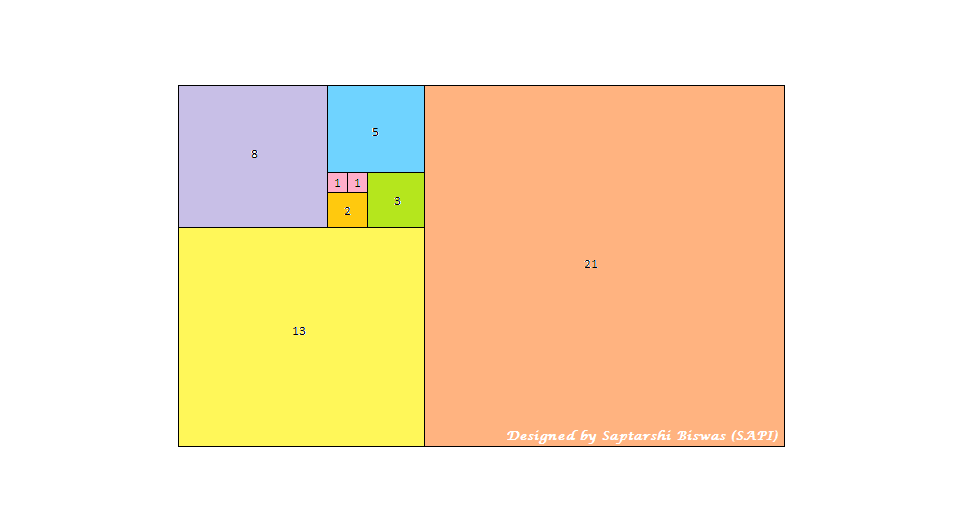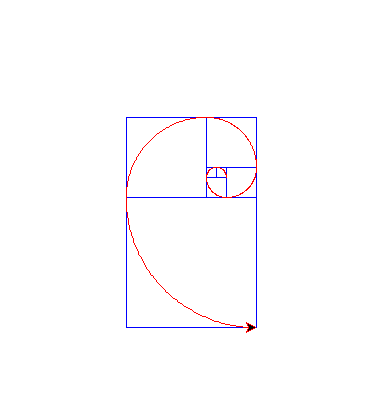# Python | Plotting Fibonacci spiral fractal using Turtle

What is Fractal Geometry?
Fractal geometry is a special form of graphical representation of mathematical functions or set of numbers generated by a mathematical function. It is a recursive, detailed and infinitely self-similar set of mathematics. These geometrical representations exhibit “Unfolding Symmetry”. Unfolding symmetry is the property of geometrical structures to retain a self-similar pattern at infinitesimally small scale.

We know Fibonacci Series follows a recursive relation f(n) = f(n-1) + f(n-2), where n is the nth term in the series. Again f(0) = 0, f(1) = 1

Approach of plotting Fibonacci Fractal

Each number in the series represent the length of the sides of a square. The square of side length 0 does not exist. So we start from square of side length 1. The next square is also of side length 1.

• We first construct the two squares of dimension 1 side by side as shown in the image below.
• Then taking the joint length of the two squares we construct a third square below the two squares of dimension 1. Now the square is of the dimension 2
• Again taking the 2 squares of dimension 1, 2 respectively we construct the fourth square of dimension 3
• Although we continued this process for a small number of iterations but this process continues till infinity.After we complete drawing the squares we start with the innermost smallest square. Then we draw continuous quadrants within the squares with the side of each square as the radius.

Below is the implementaion:

 `# Python program for Plotting Fibonacci ` `# spiral fractal using Turtle ` `import` `turtle ` `import` `math ` ` `  `def` `fiboPlot(n): ` `    ``a ``=` `0` `    ``b ``=` `1` `    ``square_a ``=` `a ` `    ``square_b ``=` `b ` ` `  `    ``# Setting the colour of the plotting pen to blue ` `    ``x.pencolor(``"blue"``) ` ` `  `    ``# Drawing the first square ` `    ``x.forward(b ``*` `factor) ` `    ``x.left(``90``) ` `    ``x.forward(b ``*` `factor) ` `    ``x.left(``90``) ` `    ``x.forward(b ``*` `factor) ` `    ``x.left(``90``) ` `    ``x.forward(b ``*` `factor) ` ` `  `    ``# Proceeding in the Fibonacci Series ` `    ``temp ``=` `square_b ` `    ``square_b ``=` `square_b ``+` `square_a ` `    ``square_a ``=` `temp ` `     `  `    ``# Drawing the rest of the squares ` `    ``for` `i ``in` `range``(``1``, n): ` `        ``x.backward(square_a ``*` `factor) ` `        ``x.right(``90``) ` `        ``x.forward(square_b ``*` `factor) ` `        ``x.left(``90``) ` `        ``x.forward(square_b ``*` `factor) ` `        ``x.left(``90``) ` `        ``x.forward(square_b ``*` `factor) ` ` `  `        ``# Proceeding in the Fibonacci Series ` `        ``temp ``=` `square_b ` `        ``square_b ``=` `square_b ``+` `square_a ` `        ``square_a ``=` `temp ` ` `  `    ``# Bringing the pen to starting point of the spiral plot ` `    ``x.penup() ` `    ``x.setposition(factor, ``0``) ` `    ``x.seth(``0``) ` `    ``x.pendown() ` ` `  `    ``# Setting the colour of the plotting pen to red ` `    ``x.pencolor(``"red"``) ` ` `  `    ``# Fibonacci Spiral Plot ` `    ``x.left(``90``) ` `    ``for` `i ``in` `range``(n): ` `        ``print``(b) ` `        ``fdwd ``=` `math.pi ``*` `b ``*` `factor ``/` `2` `        ``fdwd ``/``=` `90` `        ``for` `j ``in` `range``(``90``): ` `            ``x.forward(fdwd) ` `            ``x.left(``1``) ` `        ``temp ``=` `a ` `        ``a ``=` `b ` `        ``b ``=` `temp ``+` `b ` ` `  ` `  `# Here 'factor' signifies the multiplicative  ` `# factor which expands or shrinks the scale ` `# of the plot by a certain factor. ` `factor ``=` `1` ` `  `# Taking Input for the number of  ` `# Iterations our Algorithm will run ` `n ``=` `int``(``input``(``'Enter the number of iterations (must be > 1): '``)) ` ` `  `# Plotting the Fibonacci Spiral Fractal  ` `# and printing the corresponding Fibonacci Number ` `if` `n > ``0``: ` `    ``print``(``"Fibonacci series for"``, n, ``"elements :"``) ` `    ``x ``=` `turtle.Turtle() ` `    ``x.speed(``100``) ` `    ``fiboPlot(n) ` `    ``turtle.done() ` `else``: ` `    ``print``(``"Number of iterations must be > 0"``) `

Output:My Personal Notes arrow_drop_upCheck out this Author's contributed articles.

If you like GeeksforGeeks and would like to contribute, you can also write an article using contribute.geeksforgeeks.org or mail your article to contribute@geeksforgeeks.org. See your article appearing on the GeeksforGeeks main page and help other Geeks.

Please Improve this article if you find anything incorrect by clicking on the "Improve Article" button below.

Improved By : SAPI

Article Tags :

Be the First to upvote.

Please write to us at contribute@geeksforgeeks.org to report any issue with the above content.